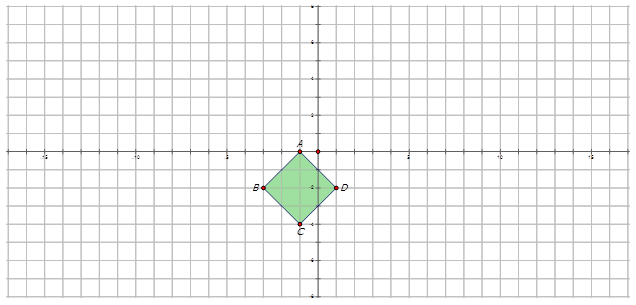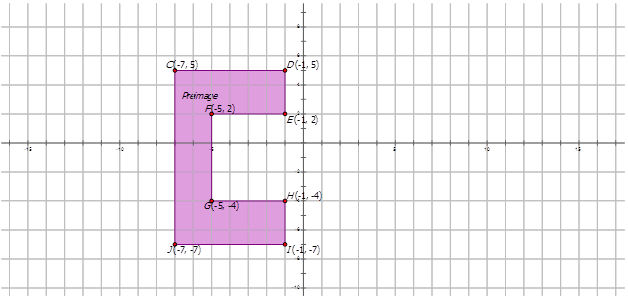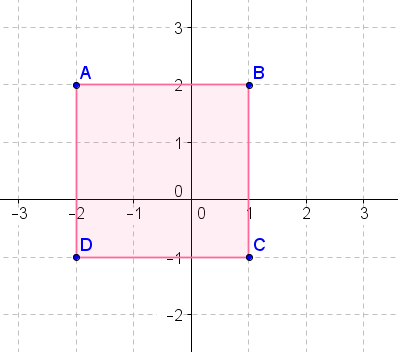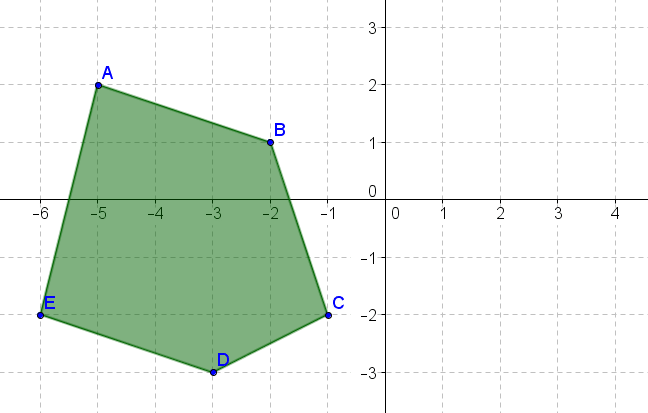# 8.12: Geometry Software and Graphing Rotations

•• Contributed by CK12
• CK12

Graph rotated images given preimage and number of degrees. Perform rotations using Geogebra.

Quadrilateral $$WXYZ$$ has coordinates $$W(−5,−5)$$, $$X(−2,0), \(Y(2,3)$$ and $$Z(−1,3)$$. Draw the quadrilateral on the Cartesian plane. Rotate the image $$110^{\circ}$$ counterclockwise about the point $$X$$. Show the resulting image.

## Graphs of Rotations

In geometry, a transformation is an operation that moves, flips, or changes a shape to create a new shape. A rotation is an example of a transformation where a figure is rotated about a specific point (called the center of rotation), a certain number of degrees.

For now, in order to graph a rotation in general you will use geometry software. This will allow you to rotate any figure any number of degrees about any point. There are a few common rotations that are good to know how to do without geometry software, shown in the table below.

Center of Rotation Angle of Rotation Preimage (Point $$P$$) Rotated Image (Point $$P′$$)
$$(0, 0)$$ $$90^{\circ}$$ (or $$−270^{\circ} ) \((x,y)$$ $$(−y,x)$$
$$(0, 0)$$ $$180^{\circ}$$ (or $$−180^{\circ}$$ ) $$(x,y)$$ $$(−x,−y)$$
$$(0, 0)$$ $$270^{\circ}$$ (or $$−90^{\circ}$$ ) $$(x,y)$$ $$(y,−x)$$

Let's draw the preimage and image and properly label each for the following transformation:

Line $$\overline{AB}$$ drawn from $$(-4, 2)$$ to $$(3, 2)$$ has been rotated about the origin at an angle of $$90^{\circ}$$ CW.Figure $$\PageIndex{1}$$

Now, let's draw and label the rotated image for the following rotations:

1. The diamond $$ABCD$$ is rotated $$145^{\circ}$$ CCW about the origin to form the image $$A′B′C′D′$$.Figure $$\PageIndex{2}$$Figure $$\PageIndex{3}$$

Notice the direction is counter-clockwise.

1. The following figure is rotated about the origin $$200^{\circ}$$ CW to make a rotated image.Figure $$\PageIndex{4}$$Figure $$\PageIndex{5}$$

Notice the direction of the rotation is counter-clockwise, therefore the angle of rotation is $$160^{\circ}$$.

Example $$\PageIndex{1}$$

Earlier, you were asked about the quadrilateral $$WXYZ$$ has coordinates $$W(−5,−5)$$, $$X(−2,0)$$, Y(2,3)\) and $$Z(−1,3)$$. Draw the quadrilateral on the Cartesian plane. Rotate the image $$110^{\circ}$$ counterclockwise about the point X\). Show the resulting image.

SolutionFigure $$\PageIndex{6}$$

Example $$\PageIndex{2}$$

Line $$\overline{ST}$$ drawn from $$(-3, 4)$$ to $$(-3, 8)$$ has been rotated $$60^{\circ}$$ CW about the point $$S$$. Draw the preimage and image and properly label each.Figure $$\PageIndex{7}$$

Solution

Notice the direction of the angle is clockwise, therefore the angle measure is $$60^{\circ}$$ CW or $$−60^{\circ}$$.

Example $$\PageIndex{3}$$

The polygon below has been rotated $$155^{\circ}$$ CCW about the origin. Draw the rotated image and properly label each.Figure $$\PageIndex{8}$$

SolutionFigure $$\PageIndex{9}$$

Notice the direction of the angle is counter-clockwise, therefore the angle measure is $$155^{\circ}$$ CCW or $$155^{\circ}$$.

Example $$\PageIndex{4}$$

The purple pentagon is rotated about the point A $$225^{\circ}$$. Find the coordinates of the purple pentagon. On the diagram, draw and label the rotated pentagon.Figure $$\PageIndex{10}$$

SolutionFigure $$\PageIndex{11}$$

The measure of $$\angle BAB′=m\angle BAE′+m\angle E′AB′$$. Therefore $$\angle BAB′=111.80^{\circ} +113.20^{\circ}$$ or $$225^{\circ}$$. Notice the direction of the angle is counter-clockwise, therefore the angle measure is $$225^{\circ}$$ CCW or $$225^{\circ}$$.

## ReviewFigure $$\PageIndex{12}$$
1. Rotate the above figure $$90^{\circ}$$ clockwise about the origin.
2. Rotate the above figure $$270^{\circ}$$ clockwise about the origin.
3. Rotate the above figure $$180^{\circ}$$ about the origin.Figure $$\PageIndex{13}$$
1. Rotate the above figure $$90^{\circ}$$ counterclockwise about the origin.
2. Rotate the above figure $$270^{\circ}$$ counterclockwise about the origin.
3. Rotate the above figure $$180^{\circ}$$ about the origin.Figure $$\PageIndex{14}$$
1. Rotate the above figure $$90^{\circ}$$ clockwise about the origin.
2. Rotate the above figure $$270^{\circ}$$ clockwise about the origin.
3. Rotate the above figure $$180^{\circ}$$ about the origin.Figure $$\PageIndex{15}$$
1. Rotate the above figure $$90^{\circ}$$ counterclockwise about the origin.
2. Rotate the above figure $$270^{\circ}$$ counterclockwise about the origin.
3. Rotate the above figure $$180^{\circ}$$ about the origin.Figure $$\PageIndex{16}$$
1. Rotate the above figure $$90^{\circ}$$ clockwise about the origin.
2. Rotate the above figure $$270^{\circ}$$ clockwise about the origin.
3. Rotate the above figure $$180^{\circ}$$ about the origin.Figure $$\PageIndex{17}$$
1. Rotate the above figure $$90^{\circ}$$ counterclockwise about the origin.
2. Rotate the above figure $$270^{\circ}$$ counterclockwise about the origin.
3. Rotate the above figure $$180^{\circ}$$ about the origin.Figure $$\PageIndex{18}$$
1. Rotate the above figure $$90^{\circ}$$ clockwise about the origin.
2. Rotate the above figure $$270^{\circ}$$ clockwise about the origin.
3. Rotate the above figure $$180^{\circ}$$ about the origin.Figure $$\PageIndex{19}$$
1. Rotate the above figure $$90^{\circ}$$ counterclockwise about the origin.
2. Rotate the above figure $$270^{\circ}$$ counterclockwise about the origin.
3. Rotate the above figure $$180^{\circ}$$ about the origin.